# ot.da¶

## Functions¶

ot.da.OT_mapping_linear(xs, xt, reg=1e-06, ws=None, wt=None, bias=True, log=False)[source]

return OT linear operator between samples

The function estimates the optimal linear operator that aligns the two empirical distributions. This is equivalent to estimating the closed form mapping between two Gaussian distributions $$N(\mu_s,\Sigma_s)$$ and $$N(\mu_t,\Sigma_t)$$ as proposed in  and discussed in remark 2.29 in .

The linear operator from source to target $$M$$

$M(x)=Ax+b$

where :

$A=\Sigma_s^{-1/2}(\Sigma_s^{1/2}\Sigma_t\Sigma_s^{1/2})^{1/2} \Sigma_s^{-1/2}$
$b=\mu_t-A\mu_s$
Parameters
• xs (np.ndarray (ns,d)) – samples in the source domain

• xt (np.ndarray (nt,d)) – samples in the target domain

• reg (float,optional) – regularization added to the diagonals of convariances (>0)

• ws (np.ndarray (ns,1), optional) – weights for the source samples

• wt (np.ndarray (ns,1), optional) – weights for the target samples

• bias (boolean, optional) – estimate bias b else b=0 (default:True)

• log (bool, optional) – record log if True

Returns

• A ((d x d) ndarray) – Linear operator

• b ((1 x d) ndarray) – bias

• log (dict) – log dictionary return only if log==True in parameters

References

14

Knott, M. and Smith, C. S. “On the optimal mapping of distributions”, Journal of Optimization Theory and Applications Vol 43, 1984

15

Peyré, G., & Cuturi, M. (2017). “Computational Optimal Transport”, 2018.

### Examples using ot.da.OT_mapping_linear¶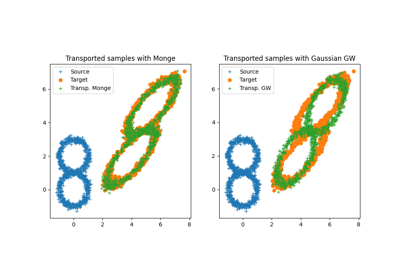ot.da.distribution_estimation_uniform(X)[source]

estimates a uniform distribution from an array of samples X

Parameters

X (array-like, shape (n_samples, n_features)) – The array of samples

Returns

mu – The uniform distribution estimated from X

Return type

array-like, shape (n_samples,)

ot.da.emd_laplace(a, b, xs, xt, M, sim='knn', sim_param=None, reg='pos', eta=1, alpha=0.5, numItermax=100, stopThr=1e-09, numInnerItermax=100000, stopInnerThr=1e-09, log=False, verbose=False)[source]

Solve the optimal transport problem (OT) with Laplacian regularization

\begin{align}\begin{aligned}\gamma = arg\min_\gamma <\gamma,M>_F + eta\Omega_\alpha(\gamma)\\s.t.\ \gamma 1 = a\\ \gamma^T 1= b\\ \gamma\geq 0\end{aligned}\end{align}

where:

• a and b are source and target weights (sum to 1)

• xs and xt are source and target samples

• M is the (ns,nt) metric cost matrix

• $$\Omega_\alpha$$ is the Laplacian regularization term $$\Omega_\alpha = (1-\alpha)/n_s^2\sum_{i,j}S^s_{i,j}\|T(\mathbf{x}^s_i)-T(\mathbf{x}^s_j)\|^2+\alpha/n_t^2\sum_{i,j}S^t_{i,j}^'\|T(\mathbf{x}^t_i)-T(\mathbf{x}^t_j)\|^2$$ with $$S^s_{i,j}, S^t_{i,j}$$ denoting source and target similarity matrices and $$T(\cdot)$$ being a barycentric mapping

The algorithm used for solving the problem is the conditional gradient algorithm as proposed in .

Parameters
• a (np.ndarray (ns,)) – samples weights in the source domain

• b (np.ndarray (nt,)) – samples weights in the target domain

• xs (np.ndarray (ns,d)) – samples in the source domain

• xt (np.ndarray (nt,d)) – samples in the target domain

• M (np.ndarray (ns,nt)) – loss matrix

• sim (string, optional) – Type of similarity (‘knn’ or ‘gauss’) used to construct the Laplacian.

• sim_param (int or float, optional) – Parameter (number of the nearest neighbors for sim=’knn’ or bandwidth for sim=’gauss’) used to compute the Laplacian.

• reg (string) – Type of Laplacian regularization

• eta (float) – Regularization term for Laplacian regularization

• alpha (float) – Regularization term for source domain’s importance in regularization

• numItermax (int, optional) – Max number of iterations

• stopThr (float, optional) – Stop threshold on error (inner emd solver) (>0)

• numInnerItermax (int, optional) – Max number of iterations (inner CG solver)

• stopInnerThr (float, optional) – Stop threshold on error (inner CG solver) (>0)

• verbose (bool, optional) – Print information along iterations

• log (bool, optional) – record log if True

Returns

• gamma ((ns x nt) ndarray) – Optimal transportation matrix for the given parameters

• log (dict) – log dictionary return only if log==True in parameters

References

5

N. Courty; R. Flamary; D. Tuia; A. Rakotomamonjy, “Optimal Transport for Domain Adaptation,” in IEEE Transactions on Pattern Analysis and Machine Intelligence , vol.PP, no.99, pp.1-1

30

R. Flamary, N. Courty, D. Tuia, A. Rakotomamonjy, “Optimal transport with Laplacian regularization: Applications to domain adaptation and shape matching,”

in NIPS Workshop on Optimal Transport and Machine Learning OTML, 2014.

ot.lp.emd

Unregularized OT

ot.optim.cg

General regularized OT

ot.da.joint_OT_mapping_kernel(xs, xt, mu=1, eta=0.001, kerneltype='gaussian', sigma=1, bias=False, verbose=False, verbose2=False, numItermax=100, numInnerItermax=10, stopInnerThr=1e-06, stopThr=1e-05, log=False, **kwargs)[source]

Joint OT and nonlinear mapping estimation with kernels as proposed in 

The function solves the following optimization problem:

\begin{align}\begin{aligned}\min_{\gamma,L\in\mathcal{H}}\quad \|L(X_s) - n_s\gamma X_t\|^2_F + \mu<\gamma,M>_F + \eta \|L\|^2_\mathcal{H}\\s.t. \gamma 1 = a\\ \gamma^T 1= b\\ \gamma\geq 0\end{aligned}\end{align}

where :

• M is the (ns,nt) squared euclidean cost matrix between samples in Xs and Xt (scaled by ns)

• $$L$$ is a ns x d linear operator on a kernel matrix that approximates the barycentric mapping

• a and b are uniform source and target weights

The problem consist in solving jointly an optimal transport matrix $$\gamma$$ and the nonlinear mapping that fits the barycentric mapping $$n_s\gamma X_t$$.

One can also estimate a mapping with constant bias (see supplementary material of ) using the bias optional argument.

The algorithm used for solving the problem is the block coordinate descent that alternates between updates of G (using conditionnal gradient) and the update of L using a classical kernel least square solver.

Parameters
• xs (np.ndarray (ns,d)) – samples in the source domain

• xt (np.ndarray (nt,d)) – samples in the target domain

• mu (float,optional) – Weight for the linear OT loss (>0)

• eta (float, optional) – Regularization term for the linear mapping L (>0)

• kerneltype (str,optional) – kernel used by calling function ot.utils.kernel (gaussian by default)

• sigma (float, optional) – Gaussian kernel bandwidth.

• bias (bool,optional) – Estimate linear mapping with constant bias

• verbose (bool, optional) – Print information along iterations

• verbose2 (bool, optional) – Print information along iterations

• numItermax (int, optional) – Max number of BCD iterations

• numInnerItermax (int, optional) – Max number of iterations (inner CG solver)

• stopInnerThr (float, optional) – Stop threshold on error (inner CG solver) (>0)

• stopThr (float, optional) – Stop threshold on relative loss decrease (>0)

• log (bool, optional) – record log if True

Returns

• gamma ((ns x nt) ndarray) – Optimal transportation matrix for the given parameters

• L ((ns x d) ndarray) – Nonlinear mapping matrix (ns+1 x d if bias)

• log (dict) – log dictionary return only if log==True in parameters

References

8

M. Perrot, N. Courty, R. Flamary, A. Habrard, “Mapping estimation for discrete optimal transport”, Neural Information Processing Systems (NIPS), 2016.

ot.lp.emd

Unregularized OT

ot.optim.cg

General regularized OT

ot.da.joint_OT_mapping_linear(xs, xt, mu=1, eta=0.001, bias=False, verbose=False, verbose2=False, numItermax=100, numInnerItermax=10, stopInnerThr=1e-06, stopThr=1e-05, log=False, **kwargs)[source]

Joint OT and linear mapping estimation as proposed in 

The function solves the following optimization problem:

\begin{align}\begin{aligned}\min_{\gamma,L}\quad \|L(X_s) -n_s\gamma X_t\|^2_F + \mu<\gamma,M>_F + \eta \|L -I\|^2_F\\s.t. \gamma 1 = a\\ \gamma^T 1= b\\ \gamma\geq 0\end{aligned}\end{align}

where :

• M is the (ns,nt) squared euclidean cost matrix between samples in

Xs and Xt (scaled by ns)

• $$L$$ is a dxd linear operator that approximates the barycentric mapping

• $$I$$ is the identity matrix (neutral linear mapping)

• a and b are uniform source and target weights

The problem consist in solving jointly an optimal transport matrix $$\gamma$$ and a linear mapping that fits the barycentric mapping $$n_s\gamma X_t$$.

One can also estimate a mapping with constant bias (see supplementary material of ) using the bias optional argument.

The algorithm used for solving the problem is the block coordinate descent that alternates between updates of G (using conditionnal gradient) and the update of L using a classical least square solver.

Parameters
• xs (np.ndarray (ns,d)) – samples in the source domain

• xt (np.ndarray (nt,d)) – samples in the target domain

• mu (float,optional) – Weight for the linear OT loss (>0)

• eta (float, optional) – Regularization term for the linear mapping L (>0)

• bias (bool,optional) – Estimate linear mapping with constant bias

• numItermax (int, optional) – Max number of BCD iterations

• stopThr (float, optional) – Stop threshold on relative loss decrease (>0)

• numInnerItermax (int, optional) – Max number of iterations (inner CG solver)

• stopInnerThr (float, optional) – Stop threshold on error (inner CG solver) (>0)

• verbose (bool, optional) – Print information along iterations

• log (bool, optional) – record log if True

Returns

• gamma ((ns x nt) ndarray) – Optimal transportation matrix for the given parameters

• L ((d x d) ndarray) – Linear mapping matrix (d+1 x d if bias)

• log (dict) – log dictionary return only if log==True in parameters

References

8

M. Perrot, N. Courty, R. Flamary, A. Habrard, “Mapping estimation for discrete optimal transport”, Neural Information Processing Systems (NIPS), 2016.

ot.lp.emd

Unregularized OT

ot.optim.cg

General regularized OT

ot.da.sinkhorn_l1l2_gl(a, labels_a, b, M, reg, eta=0.1, numItermax=10, numInnerItermax=200, stopInnerThr=1e-09, verbose=False, log=False)[source]

Solve the entropic regularization optimal transport problem with group lasso regularization

The function solves the following optimization problem:

\begin{align}\begin{aligned}\gamma = arg\min_\gamma <\gamma,M>_F + reg\cdot\Omega_e(\gamma)+ \eta \Omega_g(\gamma)\\s.t. \gamma 1 = a\\ \gamma^T 1= b\\ \gamma\geq 0\end{aligned}\end{align}

where :

• M is the (ns,nt) metric cost matrix

• $$\Omega_e$$ is the entropic regularization term $$\Omega_e(\gamma)=\sum_{i,j} \gamma_{i,j}\log(\gamma_{i,j})$$

• $$\Omega_g$$ is the group lasso regulaization term $$\Omega_g(\gamma)=\sum_{i,c} \|\gamma_{i,\mathcal{I}_c}\|^2$$ where $$\mathcal{I}_c$$ are the index of samples from class c in the source domain.

• a and b are source and target weights (sum to 1)

The algorithm used for solving the problem is the generalised conditional gradient as proposed in _ _

Parameters
• a (np.ndarray (ns,)) – samples weights in the source domain

• labels_a (np.ndarray (ns,)) – labels of samples in the source domain

• b (np.ndarray (nt,)) – samples in the target domain

• M (np.ndarray (ns,nt)) – loss matrix

• reg (float) – Regularization term for entropic regularization >0

• eta (float, optional) – Regularization term for group lasso regularization >0

• numItermax (int, optional) – Max number of iterations

• numInnerItermax (int, optional) – Max number of iterations (inner sinkhorn solver)

• stopInnerThr (float, optional) – Stop threshold on error (inner sinkhorn solver) (>0)

• verbose (bool, optional) – Print information along iterations

• log (bool, optional) – record log if True

Returns

• gamma ((ns x nt) ndarray) – Optimal transportation matrix for the given parameters

• log (dict) – log dictionary return only if log==True in parameters

References

5

N. Courty; R. Flamary; D. Tuia; A. Rakotomamonjy, “Optimal Transport for Domain Adaptation,” in IEEE Transactions on Pattern Analysis and Machine Intelligence , vol.PP, no.99, pp.1-1

7

Rakotomamonjy, A., Flamary, R., & Courty, N. (2015). Generalized conditional gradient: analysis of convergence and applications. arXiv preprint arXiv:1510.06567.

ot.optim.gcg

Generalized conditional gradient for OT problems

ot.da.sinkhorn_lpl1_mm(a, labels_a, b, M, reg, eta=0.1, numItermax=10, numInnerItermax=200, stopInnerThr=1e-09, verbose=False, log=False)[source]

Solve the entropic regularization optimal transport problem with nonconvex group lasso regularization

The function solves the following optimization problem:

\begin{align}\begin{aligned}\gamma = arg\min_\gamma <\gamma,M>_F + reg\cdot\Omega_e(\gamma) + \eta \Omega_g(\gamma)\\s.t. \gamma 1 = a\\ \gamma^T 1= b\\ \gamma\geq 0\end{aligned}\end{align}

where :

• M is the (ns,nt) metric cost matrix

• $$\Omega_e$$ is the entropic regularization term $$\Omega_e (\gamma)=\sum_{i,j} \gamma_{i,j}\log(\gamma_{i,j})$$

• $$\Omega_g$$ is the group lasso regularization term $$\Omega_g(\gamma)=\sum_{i,c} \|\gamma_{i,\mathcal{I}_c}\|^{1/2}_1$$ where $$\mathcal{I}_c$$ are the index of samples from class c in the source domain.

• a and b are source and target weights (sum to 1)

The algorithm used for solving the problem is the generalized conditional gradient as proposed in _ _

Parameters
• a (np.ndarray (ns,)) – samples weights in the source domain

• labels_a (np.ndarray (ns,)) – labels of samples in the source domain

• b (np.ndarray (nt,)) – samples weights in the target domain

• M (np.ndarray (ns,nt)) – loss matrix

• reg (float) – Regularization term for entropic regularization >0

• eta (float, optional) – Regularization term for group lasso regularization >0

• numItermax (int, optional) – Max number of iterations

• numInnerItermax (int, optional) – Max number of iterations (inner sinkhorn solver)

• stopInnerThr (float, optional) – Stop threshold on error (inner sinkhorn solver) (>0)

• verbose (bool, optional) – Print information along iterations

• log (bool, optional) – record log if True

Returns

• gamma ((ns x nt) ndarray) – Optimal transportation matrix for the given parameters

• log (dict) – log dictionary return only if log==True in parameters

References

5

N. Courty; R. Flamary; D. Tuia; A. Rakotomamonjy, “Optimal Transport for Domain Adaptation,” in IEEE Transactions on Pattern Analysis and Machine Intelligence , vol.PP, no.99, pp.1-1

7

Rakotomamonjy, A., Flamary, R., & Courty, N. (2015). Generalized conditional gradient: analysis of convergence and applications. arXiv preprint arXiv:1510.06567.

ot.lp.emd

Unregularized OT

ot.bregman.sinkhorn

Entropic regularized OT

ot.optim.cg

General regularized OT

## Classes¶

class ot.da.BaseTransport[source]

Base class for OTDA objects

Notes

All estimators should specify all the parameters that can be set at the class level in their __init__ as explicit keyword arguments (no *args or **kwargs).

the fit method should:

• estimate a cost matrix and store it in a cost_ attribute

• estimate a coupling matrix and store it in a coupling_

attribute - estimate distributions from source and target data and store them in mu_s and mu_t attributes - store Xs and Xt in attributes to be used later on in transform and inverse_transform methods

transform method should always get as input a Xs parameter inverse_transform method should always get as input a Xt parameter

transform_labels method should always get as input a ys parameter inverse_transform_labels method should always get as input a yt parameter

fit(Xs=None, ys=None, Xt=None, yt=None)[source]

Build a coupling matrix from source and target sets of samples (Xs, ys) and (Xt, yt)

Parameters
• Xs (array-like, shape (n_source_samples, n_features)) – The training input samples.

• ys (array-like, shape (n_source_samples,)) – The training class labels

• Xt (array-like, shape (n_target_samples, n_features)) – The training input samples.

• yt (array-like, shape (n_target_samples,)) –

The class labels. If some target samples are unlabeled, fill the yt’s elements with -1.

Warning: Note that, due to this convention -1 cannot be used as a class label

Returns

self – Returns self.

Return type

object

fit_transform(Xs=None, ys=None, Xt=None, yt=None)[source]

Build a coupling matrix from source and target sets of samples (Xs, ys) and (Xt, yt) and transports source samples Xs onto target ones Xt

Parameters
• Xs (array-like, shape (n_source_samples, n_features)) – The training input samples.

• ys (array-like, shape (n_source_samples,)) – The class labels for training samples

• Xt (array-like, shape (n_target_samples, n_features)) – The training input samples.

• yt (array-like, shape (n_target_samples,)) –

The class labels. If some target samples are unlabeled, fill the yt’s elements with -1.

Warning: Note that, due to this convention -1 cannot be used as a class label

Returns

transp_Xs – The source samples samples.

Return type

array-like, shape (n_source_samples, n_features)

inverse_transform(Xs=None, ys=None, Xt=None, yt=None, batch_size=128)[source]

Transports target samples Xt onto source samples Xs

Parameters
• Xs (array-like, shape (n_source_samples, n_features)) – The source input samples.

• ys (array-like, shape (n_source_samples,)) – The source class labels

• Xt (array-like, shape (n_target_samples, n_features)) – The target input samples.

• yt (array-like, shape (n_target_samples,)) –

The target class labels. If some target samples are unlabeled, fill the yt’s elements with -1.

Warning: Note that, due to this convention -1 cannot be used as a class label

• batch_size (int, optional (default=128)) – The batch size for out of sample inverse transform

Returns

transp_Xt – The transported target samples.

Return type

array-like, shape (n_source_samples, n_features)

inverse_transform_labels(yt=None)[source]

Propagate target labels yt to obtain estimated source labels ys

Parameters

yt (array-like, shape (n_target_samples,)) –

Returns

transp_ys – Estimated soft source labels.

Return type

array-like, shape (n_source_samples, nb_classes)

transform(Xs=None, ys=None, Xt=None, yt=None, batch_size=128)[source]

Transports source samples Xs onto target ones Xt

Parameters
• Xs (array-like, shape (n_source_samples, n_features)) – The source input samples.

• ys (array-like, shape (n_source_samples,)) – The class labels for source samples

• Xt (array-like, shape (n_target_samples, n_features)) – The target input samples.

• yt (array-like, shape (n_target_samples,)) –

The class labels for target. If some target samples are unlabeled, fill the yt’s elements with -1.

Warning: Note that, due to this convention -1 cannot be used as a class label

• batch_size (int, optional (default=128)) – The batch size for out of sample inverse transform

Returns

transp_Xs – The transport source samples.

Return type

array-like, shape (n_source_samples, n_features)

transform_labels(ys=None)[source]

Propagate source labels ys to obtain estimated target labels as in 

Parameters

ys (array-like, shape (n_source_samples,)) – The source class labels

Returns

transp_ys – Estimated soft target labels.

Return type

array-like, shape (n_target_samples, nb_classes)

References

27

Ievgen Redko, Nicolas Courty, Rémi Flamary, Devis Tuia “Optimal transport for multi-source domain adaptation under target shift”, International Conference on Artificial Intelligence and Statistics (AISTATS), 2019.

### Examples using ot.da.BaseTransport¶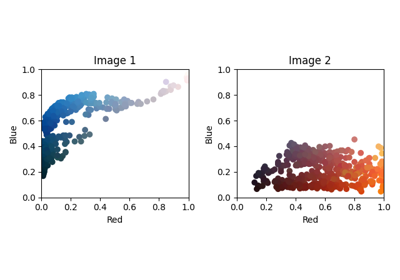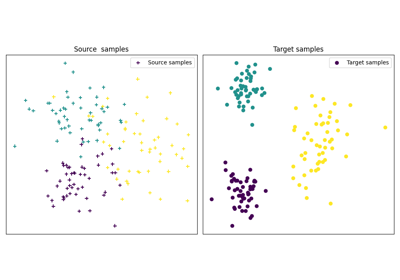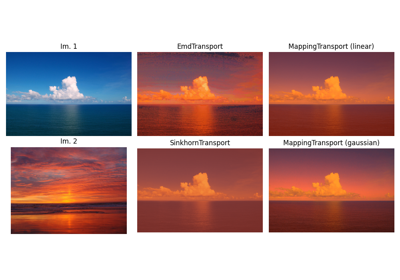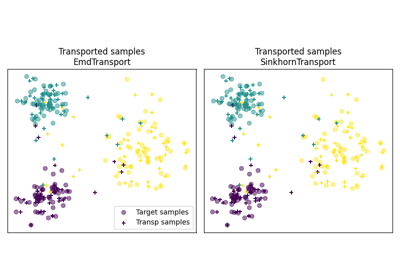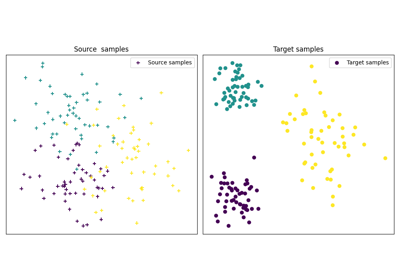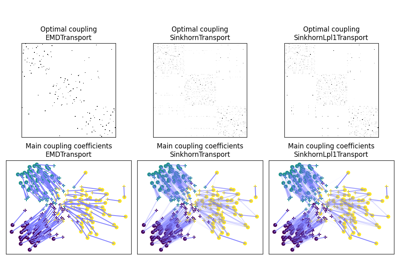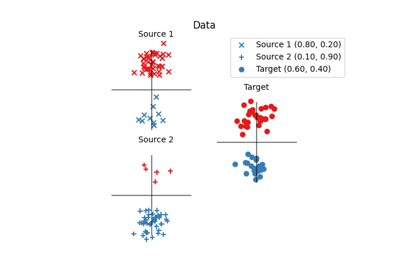class ot.da.EMDLaplaceTransport(reg_type='pos', reg_lap=1.0, reg_src=1.0, metric='sqeuclidean', norm=None, similarity='knn', similarity_param=None, max_iter=100, tol=1e-09, max_inner_iter=100000, inner_tol=1e-09, log=False, verbose=False, distribution_estimation=<function distribution_estimation_uniform>, out_of_sample_map='ferradans')[source]

Domain Adapatation OT method based on Earth Mover’s Distance with Laplacian regularization

Parameters
• reg_type (string optional (default='pos')) – Type of the regularization term: ‘pos’ and ‘disp’ for regularization term defined in  and , respectively.

• reg_lap (float, optional (default=1)) – Laplacian regularization parameter

• reg_src (float, optional (default=0.5)) – Source relative importance in regularization

• metric (string, optional (default="sqeuclidean")) – The ground metric for the Wasserstein problem

• norm (string, optional (default=None)) – If given, normalize the ground metric to avoid numerical errors that can occur with large metric values.

• similarity (string, optional (default="knn")) – The similarity to use either knn or gaussian

• similarity_param (int or float, optional (default=None)) – Parameter for the similarity: number of nearest neighbors or bandwidth if similarity=”knn” or “gaussian”, respectively. If None is provided, it is set to 3 or the average pairwise squared Euclidean distance, respectively.

• max_iter (int, optional (default=100)) – Max number of BCD iterations

• tol (float, optional (default=1e-5)) – Stop threshold on relative loss decrease (>0)

• max_inner_iter (int, optional (default=10)) – Max number of iterations (inner CG solver)

• inner_tol (float, optional (default=1e-6)) – Stop threshold on error (inner CG solver) (>0)

• log (int, optional (default=False)) – Controls the logs of the optimization algorithm

• distribution_estimation (callable, optional (defaults to the uniform)) – The kind of distribution estimation to employ

• out_of_sample_map (string, optional (default="ferradans")) – The kind of out of sample mapping to apply to transport samples from a domain into another one. Currently the only possible option is “ferradans” which uses the method proposed in .

coupling_

The optimal coupling

Type

array-like, shape (n_source_samples, n_target_samples)

References

1

N. Courty; R. Flamary; D. Tuia; A. Rakotomamonjy, “Optimal Transport for Domain Adaptation,” in IEEE Transactions on Pattern Analysis and Machine Intelligence , vol.PP, no.99, pp.1-1

2

R. Flamary, N. Courty, D. Tuia, A. Rakotomamonjy, “Optimal transport with Laplacian regularization: Applications to domain adaptation and shape matching,”

in NIPS Workshop on Optimal Transport and Machine Learning OTML, 2014.

6

Ferradans, S., Papadakis, N., Peyré, G., & Aujol, J. F. (2014). Regularized discrete optimal transport. SIAM Journal on Imaging Sciences, 7(3), 1853-1882.

fit(Xs, ys=None, Xt=None, yt=None)[source]

Build a coupling matrix from source and target sets of samples (Xs, ys) and (Xt, yt)

Parameters
• Xs (array-like, shape (n_source_samples, n_features)) – The training input samples.

• ys (array-like, shape (n_source_samples,)) – The class labels

• Xt (array-like, shape (n_target_samples, n_features)) – The training input samples.

• yt (array-like, shape (n_target_samples,)) –

The class labels. If some target samples are unlabeled, fill the yt’s elements with -1.

Warning: Note that, due to this convention -1 cannot be used as a class label

Returns

self – Returns self.

Return type

object

### Examples using ot.da.EMDLaplaceTransport¶class ot.da.EMDTransport(metric='sqeuclidean', norm=None, log=False, distribution_estimation=<function distribution_estimation_uniform>, out_of_sample_map='ferradans', limit_max=10, max_iter=100000)[source]

Domain Adapatation OT method based on Earth Mover’s Distance

Parameters
• metric (string, optional (default="sqeuclidean")) – The ground metric for the Wasserstein problem

• norm (string, optional (default=None)) – If given, normalize the ground metric to avoid numerical errors that can occur with large metric values.

• log (int, optional (default=False)) – Controls the logs of the optimization algorithm

• distribution_estimation (callable, optional (defaults to the uniform)) – The kind of distribution estimation to employ

• out_of_sample_map (string, optional (default="ferradans")) – The kind of out of sample mapping to apply to transport samples from a domain into another one. Currently the only possible option is “ferradans” which uses the method proposed in .

• limit_max (float, optional (default=10)) – Controls the semi supervised mode. Transport between labeled source and target samples of different classes will exhibit an infinite cost (10 times the maximum value of the cost matrix)

• max_iter (int, optional (default=100000)) – The maximum number of iterations before stopping the optimization algorithm if it has not converged.

coupling_

The optimal coupling

Type

array-like, shape (n_source_samples, n_target_samples)

References

1

N. Courty; R. Flamary; D. Tuia; A. Rakotomamonjy, “Optimal Transport for Domain Adaptation,” in IEEE Transactions on Pattern Analysis and Machine Intelligence , vol.PP, no.99, pp.1-1

6

Ferradans, S., Papadakis, N., Peyré, G., & Aujol, J. F. (2014). Regularized discrete optimal transport. SIAM Journal on Imaging Sciences, 7(3), 1853-1882.

fit(Xs, ys=None, Xt=None, yt=None)[source]

Build a coupling matrix from source and target sets of samples (Xs, ys) and (Xt, yt)

Parameters
• Xs (array-like, shape (n_source_samples, n_features)) – The training input samples.

• ys (array-like, shape (n_source_samples,)) – The class labels

• Xt (array-like, shape (n_target_samples, n_features)) – The training input samples.

• yt (array-like, shape (n_target_samples,)) –

The class labels. If some target samples are unlabeled, fill the yt’s elements with -1.

Warning: Note that, due to this convention -1 cannot be used as a class label

Returns

self – Returns self.

Return type

object

### Examples using ot.da.EMDTransport¶class ot.da.JCPOTTransport(reg_e=0.1, max_iter=10, tol=1e-08, verbose=False, log=False, metric='sqeuclidean', out_of_sample_map='ferradans')[source]

Domain Adapatation OT method for multi-source target shift based on Wasserstein barycenter algorithm.

Parameters
• reg_e (float, optional (default=1)) – Entropic regularization parameter

• max_iter (int, float, optional (default=10)) – The minimum number of iteration before stopping the optimization algorithm if no it has not converged

• tol (float, optional (default=10e-9)) – Stop threshold on error (inner sinkhorn solver) (>0)

• verbose (bool, optional (default=False)) – Controls the verbosity of the optimization algorithm

• log (bool, optional (default=False)) – Controls the logs of the optimization algorithm

• metric (string, optional (default="sqeuclidean")) – The ground metric for the Wasserstein problem

• norm (string, optional (default=None)) – If given, normalize the ground metric to avoid numerical errors that can occur with large metric values.

• distribution_estimation (callable, optional (defaults to the uniform)) – The kind of distribution estimation to employ

• out_of_sample_map (string, optional (default="ferradans")) – The kind of out of sample mapping to apply to transport samples from a domain into another one. Currently the only possible option is “ferradans” which uses the method proposed in .

coupling_

A set of optimal couplings between each source domain and the target domain

Type

list of array-like objects, shape K x (n_source_samples, n_target_samples)

proportions_

Estimated class proportions in the target domain

Type

array-like, shape (n_classes,)

log_

The dictionary of log, empty dic if parameter log is not True

Type

dictionary

References

1

Ievgen Redko, Nicolas Courty, Rémi Flamary, Devis Tuia “Optimal transport for multi-source domain adaptation under target shift”, International Conference on Artificial Intelligence and Statistics (AISTATS), vol. 89, p.849-858, 2019.

6

Ferradans, S., Papadakis, N., Peyré, G., & Aujol, J. F. (2014). Regularized discrete optimal transport. SIAM Journal on Imaging Sciences, 7(3), 1853-1882.

fit(Xs, ys=None, Xt=None, yt=None)[source]

Building coupling matrices from a list of source and target sets of samples (Xs, ys) and (Xt, yt)

Parameters
• Xs (list of K array-like objects, shape K x (nk_source_samples, n_features)) – A list of the training input samples.

• ys (list of K array-like objects, shape K x (nk_source_samples,)) – A list of the class labels

• Xt (array-like, shape (n_target_samples, n_features)) – The training input samples.

• yt (array-like, shape (n_target_samples,)) –

The class labels. If some target samples are unlabeled, fill the yt’s elements with -1.

Warning: Note that, due to this convention -1 cannot be used as a class label

Returns

self – Returns self.

Return type

object

inverse_transform_labels(yt=None)[source]

Propagate source labels ys to obtain target labels

Parameters

yt (array-like, shape (n_source_samples,)) – The target class labels

Returns

transp_ys – A list of estimated soft source labels

Return type

list of K array-like objects, shape K x (nk_source_samples, nb_classes)

transform(Xs=None, ys=None, Xt=None, yt=None, batch_size=128)[source]

Transports source samples Xs onto target ones Xt

Parameters
• Xs (list of K array-like objects, shape K x (nk_source_samples, n_features)) – A list of the training input samples.

• ys (list of K array-like objects, shape K x (nk_source_samples,)) – A list of the class labels

• Xt (array-like, shape (n_target_samples, n_features)) – The training input samples.

• yt (array-like, shape (n_target_samples,)) –

The class labels. If some target samples are unlabeled, fill the yt’s elements with -1.

Warning: Note that, due to this convention -1 cannot be used as a class label

• batch_size (int, optional (default=128)) – The batch size for out of sample inverse transform

transform_labels(ys=None)[source]

Propagate source labels ys to obtain target labels as in 

Parameters

ys (list of K array-like objects, shape K x (nk_source_samples,)) – A list of the class labels

Returns

yt – Estimated soft target labels.

Return type

array-like, shape (n_target_samples, nb_classes)

### Examples using ot.da.JCPOTTransport¶class ot.da.LinearTransport(reg=1e-08, bias=True, log=False, distribution_estimation=<function distribution_estimation_uniform>)[source]

OT linear operator between empirical distributions

The function estimates the optimal linear operator that aligns the two empirical distributions. This is equivalent to estimating the closed form mapping between two Gaussian distributions $$N(\mu_s,\Sigma_s)$$ and $$N(\mu_t,\Sigma_t)$$ as proposed in  and discussed in remark 2.29 in .

The linear operator from source to target $$M$$

$M(x)=Ax+b$

where :

$A=\Sigma_s^{-1/2}(\Sigma_s^{1/2}\Sigma_t\Sigma_s^{1/2})^{1/2} \Sigma_s^{-1/2}$
$b=\mu_t-A\mu_s$
Parameters
• reg (float,optional) – regularization added to the daigonals of convariances (>0)

• bias (boolean, optional) – estimate bias b else b=0 (default:True)

• log (bool, optional) – record log if True

References

14

Knott, M. and Smith, C. S. “On the optimal mapping of distributions”, Journal of Optimization Theory and Applications Vol 43, 1984

15

Peyré, G., & Cuturi, M. (2017). “Computational Optimal Transport”, 2018.

fit(Xs=None, ys=None, Xt=None, yt=None)[source]

Build a coupling matrix from source and target sets of samples (Xs, ys) and (Xt, yt)

Parameters
• Xs (array-like, shape (n_source_samples, n_features)) – The training input samples.

• ys (array-like, shape (n_source_samples,)) – The class labels

• Xt (array-like, shape (n_target_samples, n_features)) – The training input samples.

• yt (array-like, shape (n_target_samples,)) –

The class labels. If some target samples are unlabeled, fill the yt’s elements with -1.

Warning: Note that, due to this convention -1 cannot be used as a class label

Returns

self – Returns self.

Return type

object

inverse_transform(Xs=None, ys=None, Xt=None, yt=None, batch_size=128)[source]

Transports target samples Xt onto target samples Xs

Parameters
• Xs (array-like, shape (n_source_samples, n_features)) – The training input samples.

• ys (array-like, shape (n_source_samples,)) – The class labels

• Xt (array-like, shape (n_target_samples, n_features)) – The training input samples.

• yt (array-like, shape (n_target_samples,)) –

The class labels. If some target samples are unlabeled, fill the yt’s elements with -1.

Warning: Note that, due to this convention -1 cannot be used as a class label

• batch_size (int, optional (default=128)) – The batch size for out of sample inverse transform

Returns

transp_Xt – The transported target samples.

Return type

array-like, shape (n_source_samples, n_features)

transform(Xs=None, ys=None, Xt=None, yt=None, batch_size=128)[source]

Transports source samples Xs onto target ones Xt

Parameters
• Xs (array-like, shape (n_source_samples, n_features)) – The training input samples.

• ys (array-like, shape (n_source_samples,)) – The class labels

• Xt (array-like, shape (n_target_samples, n_features)) – The training input samples.

• yt (array-like, shape (n_target_samples,)) –

The class labels. If some target samples are unlabeled, fill the yt’s elements with -1.

Warning: Note that, due to this convention -1 cannot be used as a class label

• batch_size (int, optional (default=128)) – The batch size for out of sample inverse transform

Returns

transp_Xs – The transport source samples.

Return type

array-like, shape (n_source_samples, n_features)

### Examples using ot.da.LinearTransport¶class ot.da.MappingTransport(mu=1, eta=0.001, bias=False, metric='sqeuclidean', norm=None, kernel='linear', sigma=1, max_iter=100, tol=1e-05, max_inner_iter=10, inner_tol=1e-06, log=False, verbose=False, verbose2=False)[source]

MappingTransport: DA methods that aims at jointly estimating a optimal transport coupling and the associated mapping

Parameters
• mu (float, optional (default=1)) – Weight for the linear OT loss (>0)

• eta (float, optional (default=0.001)) – Regularization term for the linear mapping L (>0)

• bias (bool, optional (default=False)) – Estimate linear mapping with constant bias

• metric (string, optional (default="sqeuclidean")) – The ground metric for the Wasserstein problem

• norm (string, optional (default=None)) – If given, normalize the ground metric to avoid numerical errors that can occur with large metric values.

• kernel (string, optional (default="linear")) – The kernel to use either linear or gaussian

• sigma (float, optional (default=1)) – The gaussian kernel parameter

• max_iter (int, optional (default=100)) – Max number of BCD iterations

• tol (float, optional (default=1e-5)) – Stop threshold on relative loss decrease (>0)

• max_inner_iter (int, optional (default=10)) – Max number of iterations (inner CG solver)

• inner_tol (float, optional (default=1e-6)) – Stop threshold on error (inner CG solver) (>0)

• log (bool, optional (default=False)) – record log if True

• verbose (bool, optional (default=False)) – Print information along iterations

• verbose2 (bool, optional (default=False)) – Print information along iterations

coupling_

The optimal coupling

Type

array-like, shape (n_source_samples, n_target_samples)

mapping_

(if bias) for kernel == linear The associated mapping array-like, shape (n_source_samples (+ 1), n_features) (if bias) for kernel == gaussian

Type

array-like, shape (n_features (+ 1), n_features)

log_

The dictionary of log, empty dic if parameter log is not True

Type

dictionary

References

8

M. Perrot, N. Courty, R. Flamary, A. Habrard, “Mapping estimation for discrete optimal transport”, Neural Information Processing Systems (NIPS), 2016.

fit(Xs=None, ys=None, Xt=None, yt=None)[source]

Builds an optimal coupling and estimates the associated mapping from source and target sets of samples (Xs, ys) and (Xt, yt)

Parameters
• Xs (array-like, shape (n_source_samples, n_features)) – The training input samples.

• ys (array-like, shape (n_source_samples,)) – The class labels

• Xt (array-like, shape (n_target_samples, n_features)) – The training input samples.

• yt (array-like, shape (n_target_samples,)) –

The class labels. If some target samples are unlabeled, fill the yt’s elements with -1.

Warning: Note that, due to this convention -1 cannot be used as a class label

Returns

self – Returns self

Return type

object

transform(Xs)[source]

Transports source samples Xs onto target ones Xt

Parameters

Xs (array-like, shape (n_source_samples, n_features)) – The training input samples.

Returns

transp_Xs – The transport source samples.

Return type

array-like, shape (n_source_samples, n_features)

### Examples using ot.da.MappingTransport¶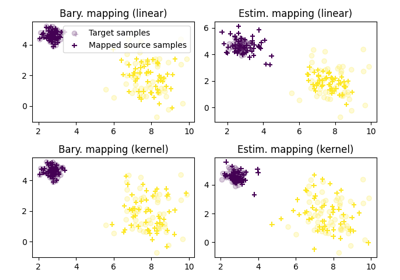class ot.da.SinkhornL1l2Transport(reg_e=1.0, reg_cl=0.1, max_iter=10, max_inner_iter=200, tol=1e-08, verbose=False, log=False, metric='sqeuclidean', norm=None, distribution_estimation=<function distribution_estimation_uniform>, out_of_sample_map='ferradans', limit_max=10)[source]

Domain Adapatation OT method based on sinkhorn algorithm + l1l2 class regularization.

Parameters
• reg_e (float, optional (default=1)) – Entropic regularization parameter

• reg_cl (float, optional (default=0.1)) – Class regularization parameter

• max_iter (int, float, optional (default=10)) – The minimum number of iteration before stopping the optimization algorithm if no it has not converged

• max_inner_iter (int, float, optional (default=200)) – The number of iteration in the inner loop

• tol (float, optional (default=10e-9)) – Stop threshold on error (inner sinkhorn solver) (>0)

• verbose (bool, optional (default=False)) – Controls the verbosity of the optimization algorithm

• log (bool, optional (default=False)) – Controls the logs of the optimization algorithm

• metric (string, optional (default="sqeuclidean")) – The ground metric for the Wasserstein problem

• norm (string, optional (default=None)) – If given, normalize the ground metric to avoid numerical errors that can occur with large metric values.

• distribution_estimation (callable, optional (defaults to the uniform)) – The kind of distribution estimation to employ

• out_of_sample_map (string, optional (default="ferradans")) – The kind of out of sample mapping to apply to transport samples from a domain into another one. Currently the only possible option is “ferradans” which uses the method proposed in .

• limit_max (float, optional (default=10)) – Controls the semi supervised mode. Transport between labeled source and target samples of different classes will exhibit an infinite cost (10 times the maximum value of the cost matrix)

coupling_

The optimal coupling

Type

array-like, shape (n_source_samples, n_target_samples)

log_

The dictionary of log, empty dic if parameter log is not True

Type

dictionary

References

1

N. Courty; R. Flamary; D. Tuia; A. Rakotomamonjy, “Optimal Transport for Domain Adaptation,” in IEEE Transactions on Pattern Analysis and Machine Intelligence , vol.PP, no.99, pp.1-1

2

Rakotomamonjy, A., Flamary, R., & Courty, N. (2015). Generalized conditional gradient: analysis of convergence and applications. arXiv preprint arXiv:1510.06567.

6

Ferradans, S., Papadakis, N., Peyré, G., & Aujol, J. F. (2014). Regularized discrete optimal transport. SIAM Journal on Imaging Sciences, 7(3), 1853-1882.

fit(Xs, ys=None, Xt=None, yt=None)[source]

Build a coupling matrix from source and target sets of samples (Xs, ys) and (Xt, yt)

Parameters
• Xs (array-like, shape (n_source_samples, n_features)) – The training input samples.

• ys (array-like, shape (n_source_samples,)) – The class labels

• Xt (array-like, shape (n_target_samples, n_features)) – The training input samples.

• yt (array-like, shape (n_target_samples,)) –

The class labels. If some target samples are unlabeled, fill the yt’s elements with -1.

Warning: Note that, due to this convention -1 cannot be used as a class label

Returns

self – Returns self.

Return type

object

### Examples using ot.da.SinkhornL1l2Transport¶class ot.da.SinkhornLpl1Transport(reg_e=1.0, reg_cl=0.1, max_iter=10, max_inner_iter=200, log=False, tol=1e-08, verbose=False, metric='sqeuclidean', norm=None, distribution_estimation=<function distribution_estimation_uniform>, out_of_sample_map='ferradans', limit_max=inf)[source]

Domain Adapatation OT method based on sinkhorn algorithm + LpL1 class regularization.

Parameters
• reg_e (float, optional (default=1)) – Entropic regularization parameter

• reg_cl (float, optional (default=0.1)) – Class regularization parameter

• max_iter (int, float, optional (default=10)) – The minimum number of iteration before stopping the optimization algorithm if no it has not converged

• max_inner_iter (int, float, optional (default=200)) – The number of iteration in the inner loop

• log (bool, optional (default=False)) – Controls the logs of the optimization algorithm

• tol (float, optional (default=10e-9)) – Stop threshold on error (inner sinkhorn solver) (>0)

• verbose (bool, optional (default=False)) – Controls the verbosity of the optimization algorithm

• metric (string, optional (default="sqeuclidean")) – The ground metric for the Wasserstein problem

• norm (string, optional (default=None)) – If given, normalize the ground metric to avoid numerical errors that can occur with large metric values.

• distribution_estimation (callable, optional (defaults to the uniform)) – The kind of distribution estimation to employ

• out_of_sample_map (string, optional (default="ferradans")) – The kind of out of sample mapping to apply to transport samples from a domain into another one. Currently the only possible option is “ferradans” which uses the method proposed in .

• limit_max (float, optional (defaul=np.infty)) – Controls the semi supervised mode. Transport between labeled source and target samples of different classes will exhibit a cost defined by limit_max.

coupling_

The optimal coupling

Type

array-like, shape (n_source_samples, n_target_samples)

References

1

N. Courty; R. Flamary; D. Tuia; A. Rakotomamonjy, “Optimal Transport for Domain Adaptation,” in IEEE Transactions on Pattern Analysis and Machine Intelligence , vol.PP, no.99, pp.1-1

2

Rakotomamonjy, A., Flamary, R., & Courty, N. (2015). Generalized conditional gradient: analysis of convergence and applications. arXiv preprint arXiv:1510.06567.

6

Ferradans, S., Papadakis, N., Peyré, G., & Aujol, J. F. (2014). Regularized discrete optimal transport. SIAM Journal on Imaging Sciences, 7(3), 1853-1882.

fit(Xs, ys=None, Xt=None, yt=None)[source]

Build a coupling matrix from source and target sets of samples (Xs, ys) and (Xt, yt)

Parameters
• Xs (array-like, shape (n_source_samples, n_features)) – The training input samples.

• ys (array-like, shape (n_source_samples,)) – The class labels

• Xt (array-like, shape (n_target_samples, n_features)) – The training input samples.

• yt (array-like, shape (n_target_samples,)) –

The class labels. If some target samples are unlabeled, fill the yt’s elements with -1.

Warning: Note that, due to this convention -1 cannot be used as a class label

Returns

self – Returns self.

Return type

object

### Examples using ot.da.SinkhornLpl1Transport¶class ot.da.SinkhornTransport(reg_e=1.0, max_iter=1000, tol=1e-08, verbose=False, log=False, metric='sqeuclidean', norm=None, distribution_estimation=<function distribution_estimation_uniform>, out_of_sample_map='ferradans', limit_max=inf)[source]

Domain Adapatation OT method based on Sinkhorn Algorithm

Parameters
• reg_e (float, optional (default=1)) – Entropic regularization parameter

• max_iter (int, float, optional (default=1000)) – The minimum number of iteration before stopping the optimization algorithm if no it has not converged

• tol (float, optional (default=10e-9)) – The precision required to stop the optimization algorithm.

• verbose (bool, optional (default=False)) – Controls the verbosity of the optimization algorithm

• log (int, optional (default=False)) – Controls the logs of the optimization algorithm

• metric (string, optional (default="sqeuclidean")) – The ground metric for the Wasserstein problem

• norm (string, optional (default=None)) – If given, normalize the ground metric to avoid numerical errors that can occur with large metric values.

• distribution_estimation (callable, optional (defaults to the uniform)) – The kind of distribution estimation to employ

• out_of_sample_map (string, optional (default="ferradans")) – The kind of out of sample mapping to apply to transport samples from a domain into another one. Currently the only possible option is “ferradans” which uses the method proposed in .

• limit_max (float, optional (defaul=np.infty)) – Controls the semi supervised mode. Transport between labeled source and target samples of different classes will exhibit an cost defined by this variable

coupling_

The optimal coupling

Type

array-like, shape (n_source_samples, n_target_samples)

log_

The dictionary of log, empty dic if parameter log is not True

Type

dictionary

References

1

N. Courty; R. Flamary; D. Tuia; A. Rakotomamonjy, “Optimal Transport for Domain Adaptation,” in IEEE Transactions on Pattern Analysis and Machine Intelligence , vol.PP, no.99, pp.1-1

2

M. Cuturi, Sinkhorn Distances : Lightspeed Computation of Optimal Transport, Advances in Neural Information Processing Systems (NIPS) 26, 2013

6

Ferradans, S., Papadakis, N., Peyré, G., & Aujol, J. F. (2014). Regularized discrete optimal transport. SIAM Journal on Imaging Sciences, 7(3), 1853-1882.

fit(Xs=None, ys=None, Xt=None, yt=None)[source]

Build a coupling matrix from source and target sets of samples (Xs, ys) and (Xt, yt)

Parameters
• Xs (array-like, shape (n_source_samples, n_features)) – The training input samples.

• ys (array-like, shape (n_source_samples,)) – The class labels

• Xt (array-like, shape (n_target_samples, n_features)) – The training input samples.

• yt (array-like, shape (n_target_samples,)) –

The class labels. If some target samples are unlabeled, fill the yt’s elements with -1.

Warning: Note that, due to this convention -1 cannot be used as a class label

Returns

self – Returns self.

Return type

object

### Examples using ot.da.SinkhornTransport¶class ot.da.UnbalancedSinkhornTransport(reg_e=1.0, reg_m=0.1, method='sinkhorn', max_iter=10, tol=1e-09, verbose=False, log=False, metric='sqeuclidean', norm=None, distribution_estimation=<function distribution_estimation_uniform>, out_of_sample_map='ferradans', limit_max=10)[source]

Domain Adapatation unbalanced OT method based on sinkhorn algorithm

Parameters
• reg_e (float, optional (default=1)) – Entropic regularization parameter

• reg_m (float, optional (default=0.1)) – Mass regularization parameter

• method (str) – method used for the solver either ‘sinkhorn’, ‘sinkhorn_stabilized’ or ‘sinkhorn_epsilon_scaling’, see those function for specific parameters

• max_iter (int, float, optional (default=10)) – The minimum number of iteration before stopping the optimization algorithm if no it has not converged

• tol (float, optional (default=10e-9)) – Stop threshold on error (inner sinkhorn solver) (>0)

• verbose (bool, optional (default=False)) – Controls the verbosity of the optimization algorithm

• log (bool, optional (default=False)) – Controls the logs of the optimization algorithm

• metric (string, optional (default="sqeuclidean")) – The ground metric for the Wasserstein problem

• norm (string, optional (default=None)) – If given, normalize the ground metric to avoid numerical errors that can occur with large metric values.

• distribution_estimation (callable, optional (defaults to the uniform)) – The kind of distribution estimation to employ

• out_of_sample_map (string, optional (default="ferradans")) – The kind of out of sample mapping to apply to transport samples from a domain into another one. Currently the only possible option is “ferradans” which uses the method proposed in .

• limit_max (float, optional (default=10)) – Controls the semi supervised mode. Transport between labeled source and target samples of different classes will exhibit an infinite cost (10 times the maximum value of the cost matrix)

coupling_

The optimal coupling

Type

array-like, shape (n_source_samples, n_target_samples)

log_

The dictionary of log, empty dic if parameter log is not True

Type

dictionary

References

1

Chizat, L., Peyré, G., Schmitzer, B., & Vialard, F. X. (2016).

Scaling algorithms for unbalanced transport problems. arXiv preprint arXiv:1607.05816. ..  Ferradans, S., Papadakis, N., Peyré, G., & Aujol, J. F. (2014).

Regularized discrete optimal transport. SIAM Journal on Imaging Sciences, 7(3), 1853-1882.

fit(Xs, ys=None, Xt=None, yt=None)[source]

Build a coupling matrix from source and target sets of samples (Xs, ys) and (Xt, yt)

Parameters
• Xs (array-like, shape (n_source_samples, n_features)) – The training input samples.

• ys (array-like, shape (n_source_samples,)) – The class labels

• Xt (array-like, shape (n_target_samples, n_features)) – The training input samples.

• yt (array-like, shape (n_target_samples,)) –

The class labels. If some target samples are unlabeled, fill the yt’s elements with -1.

Warning: Note that, due to this convention -1 cannot be used as a class label

Returns

self – Returns self.

Return type

object